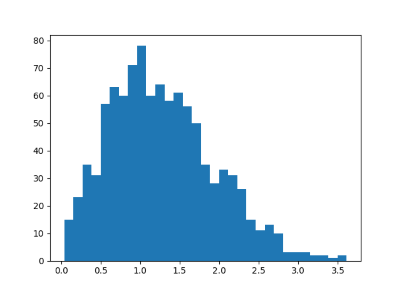Over a million developers have joined DZone.{{announcement.body}}
{{announcement.title}}

# Distribution of Eigenvalues for Gaussian Matrix

DZone 's Guide to

# Distribution of Eigenvalues for Gaussian Matrix

### In this post, we will look at the eigenvalues of matrices with some structure. And then plug this into some Python code to get data visualization.

· Big Data Zone ·
Free Resource

Comment (0)

Save
{{ articles.views | formatCount}} Views

## Symmetric Gaussian Matrices

The previous post looked at the distribution of eigenvalues for very general random matrices. In this post, we will look at the eigenvalues of matrices with more structure. Fill an n by n matrix A with values drawn from a standard normal distribution and let M be the average of A and its transpose, i.e. M = ½( A + AT). The eigenvalues will all be real because M is symmetric.

This is called a "Gaussian Orthogonal Ensemble" or GOE. The term is standard but a little misleading because such matrices may not be orthogonal.

## Eigenvalue Distribution

The joint probability distribution for the eigenvalues of M has three terms: a constant term that we will ignore, an exponential term, and a product term. ( Source)The exponential term is the same as in a multivariate normal distribution. This says the probability density drops off quickly as you go away from the origin, i.e. it's rare for eigenvalues to be too big. The product term multiplies the distances between each pair of eigenvalues. This says it's also rare for eigenvalues to be very close together.

(The missing constant to turn the expression above from a proportionality to an equation is whatever it has to be for the right side to integrate to 1. When trying to qualitatively understand a probability density, it usually helps to ignore proportionality constants. They are determined by the rest of the density expression, and they're often complicated.)

If eigenvalues are neither tightly clumped together, nor too far apart, we'd expect that the distance between them has a distribution with a hump away from zero, and a tail that decays quickly. We will demonstrate this with a simulation, then give an exact distribution.

## Python Simulation

The following Python code simulates 2-by-2 Gaussian matrices.

``````    import matplotlib.pyplot as plt
import numpy as np

n = 2
reps = 1000

diffs = np.zeros(reps)
for r in range(reps):
A = np.random.normal(scale=n**-0.5, size=(n,n))
M = 0.5*(A + A.T)
w = np.linalg.eigvalsh(M)
diffs[r] = abs(w - w)

plt.hist(diffs, bins=int(reps**0.5))
plt.show()``````

This produced the following histogram:The exact probability distribution is p( s) = s exp(- s ²/4)/2. This result is known as "Wigner's surmise."

Topics:
probability and statistics ,big data ,python ,data distribution

Comment (0)

Save
{{ articles.views | formatCount}} Views

Published at DZone with permission of

Opinions expressed by DZone contributors are their own.

# {{ parent.title || parent.header.title}}

{{ parent.tldr }}

{{ parent.urlSource.name }}